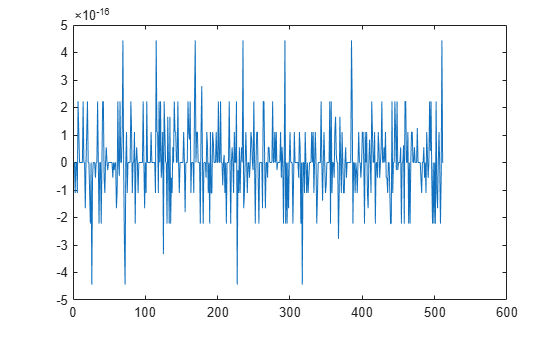# allpass2wdf

Allpass to Wave Digital Filter coefficient transformation

## Syntax

``w = allpass2wdf(a)``
``W = allpass2wdf(A)``

## Description

````w = allpass2wdf(a)` accepts a vector of real allpass polynomial filter coefficients `a`, and returns the transformed coefficient `w`. `w` can be used with allpass filter objects such as `dsp.AllpassFilter`, and `dsp.CoupledAllpassFilter`, with Structure set to `'Wave Digital Filter'`. ```
````W = allpass2wdf(A)` accepts the cell array of allpass polynomial coefficient vectors `A`. Each cell of `A` holds the coefficients of a section of a cascade allpass filter. `W` is also a cell array, and each cell of `W` contains the transformed version of the coefficients in the corresponding cell of `A`. W can be used with allpass filter objects such as `dsp.AllpassFilter` and `dsp.CoupledAllpassFilter`, with structure set to `'Wave Digital Filter'`. ```

## Examples

collapse all

Note: This example runs only in R2016b or later. If you are using an earlier release, replace each call to the function with the equivalent `step` syntax. For example, myObject(x) becomes step(myObject,x).

Create a second order allpass filter with coefficients a = [0 0.5]. Convert these coefficients into wave digital filter form using `allpass2wdf`. Assign the transformed coefficients to an allpass filter using the wave digital filter structure. Pass a random input to both these filters and compare the outputs.

```a = [0 0.5]; allpass = dsp.AllpassFilter('AllpassCoefficients', a); w = allpass2wdf(a); allpasswdf = dsp.AllpassFilter('Structure', 'Wave Digital Filter',... 'WDFCoefficients', w); in = randn(512, 1); outputAllpass = allpass(in); outputAllpasswdf = allpasswdf(in); plot(outputAllpass-outputAllpasswdf)```The difference between the two outputs is very small.

## Input Arguments

collapse all

Numeric vector of allpass filter coefficients, specified as real numbers. `a` can have length only equal to 1,2, and 4. When the length is 4, the first and third components must both be zero. `a` can be a row or a column vector.

Example: `0.7`

Data Types: `double` | `single`

Cascade of allpass filter coefficients, specified as a cell vector. Every cell of `A` must contain a real vector of length 1,2, or 4. When the length is 4, the first and third components must both be zero. `A` can be a row or column vector of cells.

Example: `{0.7, [0.1, 0.2]}`

Data Types: `double` | `single`

## Output Arguments

collapse all

Numeric vector of transformed coefficients, determined as a real number, to use with single-section allpass filter objects having `Structure` set to `'Wave Digital Filter'`. `w` is always returned as a numeric row vector.

Example: `0.7`

Data Types: `double` | `single`

Cascade of transformed allpass filter coefficients, determined as a cell array, to use with multi-section allpass filter objects having `Structure` set to ```'Wave Digital Filter'```. `W` is always returned as a column of cells.

Example: `{0.7;[0.2,-0.0833]}`

Data Types: `double` | `single`

## Algorithms

In the more general case, the input coefficients `A` define a cascade or multisection allpass filter. `allpass2wdf` applies separately to each section of the same transformation used in the single-section case. In the single-section case, the numeric coefficients vector a contains a standard polynomial representation of an allpass filter of order 1, 2, or 4. For example, in the first order case,

`$a=\left[{a}_{1}\right]$`

represents the first order transfer function:

`${H}_{1}\left(z\right)=\frac{{z}^{-1}+{a}_{1}}{1+{a}_{1}{z}^{-1}}$`

and in the second order case,

`$a=\left[{a}_{1},{a}_{2}\right]$`

represents the second order transfer function:

`${H}_{2}\left(z\right)=\frac{{z}^{-2}+{a}_{1}{z}^{-1}+{a}_{2}}{1+{a}_{1}{z}^{-1}+{a}_{2}{z}^{-2}}$`

.

The allpass transfer functions H1 and H2 can also have the following alternative representations, using decoupled coefficients in vector w1 or w2 respectively.

`${\stackrel{~}{H}}_{1}\left(z\right)=\frac{{z}^{-1}+{w}_{1}}{1+{w}_{1}{z}^{-1}}$`

`$\stackrel{}{{\stackrel{~}{H}}_{2}\left(z\right)=\frac{{z}^{-2}+{w}_{2}\left(1+{w}_{1}\right){z}^{-1}+{w}_{1}}{1+{w}_{2}\left(1+{w}_{1}\right){z}^{-1}+{w}_{1}{z}^{-2}}}$`

For allpass coefficients, w is often used to derive adaptor multipliers for Wave Digital Filter structures, and it is required by a number of allpass based filters in DSP System Toolbox™ when `Structure` is set to `'Wave Digital Filter'` (e.g. `dsp.AllpassFilter`, and `dsp.CoupledAllpassFilter`).

For a given vector of section coefficients a, `allpass2wdf` computes the corresponding vector w such that

This results in using the following formulas:

 M. Lutovac, D. Tosic, B. Evans, Filter Design for Signal Processing using MATLAB and Mathematica. Prentice Hall, 2001.